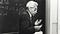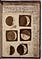# Building Abstract Thinking in Math Using Epsilon-DeltaSource: “Schedules” by Nate Armstrong on INPRNT

When I close my eyes and go back in time, I see a college student sitting in the back row and looking sad while the professor is standing next to the chalkboard, writing mathematical definitions on it with chalk. The click, click, click sound was still obvious every time the professor wrote on the blackboard. Then, the student goes into deep thought when the professor said:

`“For every epsilon greater than 0 [ε>0], there exists a delta greater than 0. [ δ>0]”`

Upon hearing this, the student asked himself repeatedly: What does epsilon mean? That student actually was me. Although everyone told me I was smart at the high school, I was just an average student at the college. The thing that made me an average student was the Greek letter “epsilon.” I could not grasp the idea of epsilon, and as a result, I struggled in Calculus for a long time. Epsilon was a nightmare for me in my college years. I felt, “What the heck! Epsilon is everywhere”. Even the arguments that I read about the epsilon simply weren’t convincing enough. I felt obscure every time I saw epsilon because it seemed bizarre to me. I was gauging my understanding of epsilon based on my scores in my Calculus exams. I was told mathematics is fun for everyone in this world, but in my case, I was unable to enjoy it because of the epsilon. Then I realized that I wasn’t the only one who had an issue understanding the epsilon. The generations before me also had a problem understanding it.

Then something extraordinary happened one autumn evening when I read a book that explained what it means. After reading the book, I fully understood what epsilon means. The book was “The Man Who Loved Only Numbers: The Story of Paul Erdos and the Search for Mathematical Truth.” The book was about Paul Erdös, the great mathematician, who called his children “epsilon.” It may seem awkward, but it was at this moment I grasped the idea of epsilon. Epsilon represents a small quantity of a number, and it is not a constant like children. Children are little, and they always change. Then I stopped cursing the concept of epsilon-delta, and I started loving it. That semester I also passed my Calculus examination, and I feel happy about it even today.Paul Erdös | Source: JustWatch

For Galileo, the old man who said “And yet it moves” just before arrested by the Church, the book of nature seemed to be written in mathematical language. Galileo was able to read and understand the language of mathematics. We know this because he was the only one in the 16th century who knew that the Earth moves around the Sun and had the courage to stand against the Church’s doctrine and say it.Galileo’s drawings of the moon, 1610 | Source: Reddit

The genius minds like Galileo discovered mathematics with their thinking, and it opened a whole different universe for them. Today young minds may struggle to learn that language, but in order to understand the language of mathematics, the only prerequisites are curiosity and imagination. Thus mathematical thinking is necessary for understanding and using ideas.

Actually, humans always think of improving their understanding of their environment. A thing, a happening, or a word can make us begin thinking. Most of these thoughts are related to mathematical thinking. For instance, when we see a group of objects, we may want to know how many.

So, epsilon is like the first step to start deep mathematical or abstract thinking. It is just another variable like x or n, and mathematicians use it in algebraic inequalities. However, it is not like pi or e and does not actually have a full definition. In the limit theory, epsilon is used to express numbers infinitely close to zero but not zero. So we can say epsilon is an “arbitrarily small positive real number,” and this is the most important thing that we need to know about epsilon. In other words, think about a positive number that is greater than only zero and less than all the positive numbers and instead of expressing it in 10 seconds every time, just call it “epsilon.” By the way, epsilon can include numbers that are far from zero when we need it. There’s nothing mathematically wrong with making epsilon stand for anything.

We can ask if the epsilon just one number or many numbers? The answer is fascinating. Yes, of course, it is only one number, but it is also not a constant number. The epsilon can represent all positive numbers. For instance, if we say 0.1 is very close to 0 and call it epsilon, we are mistaken because 0.01 is smaller than 0.1. However, 0.01 is not the smallest positive number because 0.001 is smaller than 0.01.

Similarly, 0.0001 is smaller than 0.001, and 0.00001 is smaller than 0.0001, and so on. You can keep writing “0.000000000000…” until you get close to an infinitesimally small value. Unfortunately, you will still never get the smallest positive number because there will always be …001… just after your last 0 to be smaller than your number, just like Zeno’s paradoxes. So we use epsilon to denote a small quantity, like an error.

Talk about Epsilon is not complete before we see how it is using in limits. In the first lecture on limits, we see:The limit of the function goes to L while x approaches a.
`Epsilon-Delta Proof: Given ε > 0, let δ > 0 [something positive, usually depending on ε and a]. If 0 < |x − a| < δ then |f(x) − L| < ε.`

This epsilon-delta proof is always the most famous topic in the world of calculus. It is because this proof might be the first abstract topic that has puzzled math students for centuries. We cannot blame them for it because it is not easy to grasp a mathematical definition demonstrated in a purely symbolic manner.

Interestingly, Leibniz and Newton had never used epsilon-delta proof, who almost simultaneously and independently invented the Calculus many years ago. The epsilon-delta proof is first seen in the works of Cauchy, Résumé des leçons Sur le Calcul infinitésimal, nearly 150 years after Leibniz and Newton.

The precise definition above is about the continuity of a function. Here, continuity means we can draw a graph of a function from the point x to point a without lifting the pencil. In a continuous function, when we plug a value, we always get an output. So for the inputs “x and a,” we get “f(x) and f(a)” as an input. When we make the distance |x-a| smaller and smaller horizontally, the distance |f(x) — f(a)|will be smaller and smaller vertically.

Let’s do a small example.

`f(x)= 3x+2. Then by the definition,|f(x+δ)-f(x) = |3(x+δ)+2-(3(x)+2)|=|3(δ)|. Here 3(δ)needs to be less than or equal to ε. So we can define that δ=ε/3. ThusFor ε>0, let δ<ε/3. Then |f(x+δ)-f(x)|=|3(x+δ)+2-(3(x)+2)|=|3(δ)|❤(ε/3)= ε. We are done or QED.You can find more examples here.`

We also have the concept of epsilon neighborhood in mathematics. Basically, an epsilon-neighborhood is the set of points inside an n-ball with center x and radius ε>0.

`|𝑥−𝑎|< 𝜖 ⟺ −𝜖 < 𝑥−𝑎 < 𝜖 ⟺ 𝑎−𝜖 < 𝑥 < 𝑎 + 𝜖`

This explanation is confusing, correct? Let me explain it differently. Let’s assume that you want to approach a specific value, for instance, 5. You can approach from left or right. Now, imagine that you put your five inside a bubble. It is evident that our bubble has infinitely many real numbers, and those numbers will be less than or greater than 5. If we squeeze and make our bubble smaller, we will still have infinite numbers inside the bubble around our target, 5. All we need to do is zoom-in on our bubble enough times and see infinitely many numbers while we are getting very close to 5.

`44.54.94.994.9994.99994.999994.999999……… See, infinitely many numbers between 4&5.`

So the distance between the two numbers can be made as small as we wish because the distance between those two elements is less than any number. Now we can call the distance between 5 and the border of the bubble “epsilon,” which is obviously greater than 0. Technically we can write like this;

`|x-5| < ε where x is a point inside the border. When ε=0, there will be no bubble. Source: Wikipedia`

I just want to mention one more point. If you use the idea of epsilon to impress your friend or your date, you will be wasting your time because it does not make you look cool!

## However, Mathematics

Life is good for only two things, discovering mathematics…

Written by

## Ali

Math Teacher. Content Curator. Soccer player. Maradona fan. Mostly write about the lectures I love to learn better. alikayaspor@gmail.com

## However, Mathematics

Life is good for only two things, discovering mathematics and teaching mathematics.

Written by

## Ali

Math Teacher. Content Curator. Soccer player. Maradona fan. Mostly write about the lectures I love to learn better. alikayaspor@gmail.com## However, Mathematics

Life is good for only two things, discovering mathematics and teaching mathematics.

## More From Medium

Medium is an open platform where 170 million readers come to find insightful and dynamic thinking. Here, expert and undiscovered voices alike dive into the heart of any topic and bring new ideas to the surface. Learn more

Follow the writers, publications, and topics that matter to you, and you’ll see them on your homepage and in your inbox. Explore

If you have a story to tell, knowledge to share, or a perspective to offer — welcome home. It’s easy and free to post your thinking on any topic. Write on Medium